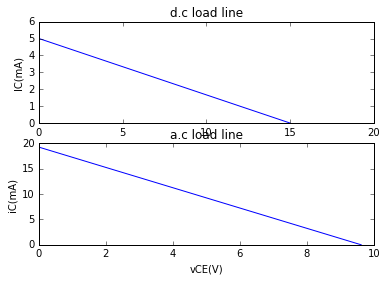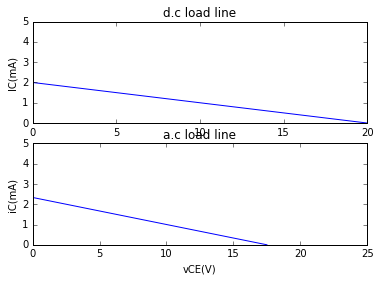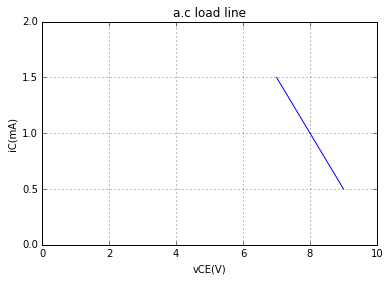# CHAPTER 10 : SINGLE STAGE TRANSISTOR AMPLIFIERS¶

## Example 10.2 : page number 243-244¶

In :
from math import pi
#Variable declaration
f_min=2.0;                            #Minimum frequency of operation of amplifier, kHz
f_max=10.0;                           #Maximum frequency of operation of amplifier, kHz
RE=560.0;                             #Emitter resistor, тДж

#Calculations
#X_CE(Emitter capacitor's capacitive reactance)
#X_CE=1/(2*pi*f_min*CE)=RE/10
#From the above equation.
CE=1/(2*pi*f_min*1000*(RE/10));              #Emitter capacitor, F,

CE=CE*10**6;                                 #Emitter capacitor, ЁЭЬЗF

#Results
print('The value of the emitter capacitor = %.2f ЁЭЬЗF'%(CE));

The value of the emitter capacitor = 1.42 ЁЭЬЗF


## Example 10.5: Page number 252-253¶

In :
%matplotlib inline
import matplotlib.pyplot as plt

#Variable declaration
VCC=15.0;                         #Collector supply voltage in V
VBE=0.7;                          #Base-emitter voltage, V
R1=10.0;                          #Resistor R1, kтДж
R2=5.0;                           #Resistor R2, kтДж
RC=1.0;                           #Collector resistor, kтДж
RE=2.0;                           #Emitter resistor, kтДж

#Calculation
#(i)
#For d.c load line, from the equation: VCE=VCC-IC*(RC+RE),
#VCE is maximum when IC=0 and IC is maximum when VCE=0.
VCE_max=VCC;                                        #Maximum collector-emitter voltage, V
IC_max=VCC/(RC+RE);                                 #Maximum collector current, mA

#plot
VCE_plot=[i for i in range(0,(int)(VCC+1))];          #Plot variable for V_CE
IC_plot=[((VCC-i)/(RC+RE)) for i in (VCE_plot[:])];      #Plot variable for I_C

plt.subplot(211)
plt.xlim(0,20)
plt.ylim(0,6)
plt.plot(VCE_plot,IC_plot);
plt.xlabel("VCE(V)");
plt.ylabel("IC(mA)");

#(ii)
#For operating point:
#Assuming VCC drops almost completely across R1 and R2,
V2=VCC*R2/(R1+R2);                                  #Voltage across resistor R2, V
IE=(V2-VBE)/RE;                                     #Emitter current, mA
IC=IE;                                              #Collector current, mA
VCE=VCC-IC*(RC+RE);                                 #Collector-emitter voltage , V

print("The operating point: VCE=%.2fV and IC=%.2fmA."%(VCE,IC));

#(iii)
VCE_ac_max=VCE+IC*RAC;                              #Maximum collector-emitter voltage, V
IC_ac_max=IC+VCE/RAC;                               #Maximum collector current, mA
print("Maximum v_CE=%.2fV and maximum i_C=%.2fmA"%(VCE_ac_max,IC_ac_max));

#plot
vCE_plot=[0,VCE_ac_max];          #Plot variable for V_CE
iC_plot=[IC_ac_max,0];      #Plot variable for I_C

plt.subplot(212)
plt.xlim(0,10)
plt.ylim(0,20)
plt.plot(vCE_plot,iC_plot);
plt.xlabel("vCE(V)");
plt.ylabel("iC(mA)");

The operating point: VCE=8.55V and IC=2.15mA.
Maximum v_CE=9.62V and maximum i_C=19.25mA## Example 10.6: Page number 253-254¶

In :
%matplotlib inline
import matplotlib.pyplot as p

#Variable declaration
RC=10;                          #Collector resistor, kтДж
VCC=20;                         #Collector supply voltage, V
IC=1;                           #Collector current, mA
VCE=10;                         #Collector-emitter voltage, V

#Calculations
#From the equation: VCE=VCC-IC*(RC+RE),
#When VCE=0, IC is  maximum.
#Emitter resistor is neglected, assuming it as negligible
IC_max=VCC/RC;                      #Maximum collector current, mA

#And, when IC=0, VCE is maximum
VCE_max=VCC;                        #Maximum collector-emitter voltage, V

#plot
p.subplot(211)
p.xlim(0,20)
p.ylim(0,5)
VCE_plot=[0,VCE_max];          #Plot variable for V_CE
IC_plot=[IC_max,0];            #Plot variable for I_C

p.plot(VCE_plot,IC_plot);
p.xlabel("VCE(V)");
p.ylabel("IC(mA)");

VCE_ac_max=VCE+IC*RAC;                 #Maximum collector-emitter voltage, V
IC_ac_max=IC+ VCE/RAC;                #Maximum collector current, mA

#plot
p.subplot(212)
p.xlim([0,25])
p.ylim([0,5])
vCE_plot=[0,VCE_ac_max];          #Plot variable for V_CE
iC_plot=[IC_ac_max,0];      #Plot variable for I_C

p.plot(vCE_plot,iC_plot);
p.xlabel("vCE(V)");
p.ylabel("iC(mA)");## Example 10.7 : Page number 254-255¶

In :
%matplotlib inline
import matplotlib.pyplot as p

#Variabe declaration
VCE_Q=8.0;           #Q-point collector emitter voltage, V
IC_Q=1;               #Q-point collector current, mA
ic_positive_peak=1.5;        #Collector current at positive peak of signal, mA
ic_negative_peak=0.5;         #Collector current at negative peak of signal, mA
vce_positive_peak=7;          #Collector emitter voltage at positive peak of signal, V
vce_negative_peak=9;          #Collector emitter voltage at negative peak of signal, V

#Plot
vce_plot=[vce_positive_peak,vce_negative_peak];    #Plot variable of vce
ic_plot=[ic_positive_peak,ic_negative_peak];    #Plot variable of ic

p.xlim(0,10)
p.ylim(0,2)
p.plot(vce_plot,ic_plot);
p.xlabel("vCE(V)");
p.ylabel("iC(mA)");
p.grid();## Example 10.8 : Page number 256¶

In :
#Variable declaration
VCC=10.0;                 #Collector supply voltage, V
RC=2.0;                   #Collector resistor, kтДж
Rin=1.0;                  #Input resistance, kтДж
beta=60.0;                #Base current amplification factor

#Calculation
Av=beta*(RAC/Rin);                  #Voltage gain

#Results
print("Voltage gain= %d."%Av);

Voltage gain= 24.


## Example 10.9 : Page number 256¶

In :
#Variable declaration
V_in=1.0;                      #Input voltage , mV
RC=10.0;                      #Collector resistor, kтДж
Rin=2.5;                      #Input resistance, kтДж
beta=100.0;                   #Base current amplification factor

#Calculations
Av=beta*(RAC/Rin);                  #Voltage gain

V_out=V_in*Av;                      #Output voltage, V

#Results
print("Output voltage= %dmV."%V_out);

Output voltage= 200mV.


## Example 10.10 : Page number 256-257¶

In :
#Variable declaration
change_in_IB=10.0;                    #Change in base current, ЁЭЬЗA
change_in_IC=1.0;                     #Change in collector current, mA
change_in_VBE=0.02;                   #Change in Base-emitter voltage, V
RC=5.0;                               #Collector resistor, kтДж
RL=10.0;                              #Emitter resistor, kтДж

#Calculations
#(i)
beta=(change_in_IC*1000)/change_in_IB;         #Base current amplification factor

#(ii)
Rin=(change_in_VBE/change_in_IB)*1000;         #Input impedance, kтДж

#(iii)

#(iv)
Av=beta*RAC/Rin;                                #Voltage gain

#(v)
Ap=beta*Av;                                     #Power gain

#Results
print("Beta= %d."%beta);
print("Input impedance=%d kтДж."%Rin);
print("Voltage gain= %d."%Av);
print("Power gain=%d."%Ap);

Beta= 100.
Input impedance=2 kтДж.
Voltage gain= 165.
Power gain=16500.


## Example 10.11 : Page number 257¶

In :
#Variable declaration
beta=50.0;                      #Base current amplification factor
RC=3.0;                         #Collector resistor,kтДж
Rin=0.5;                        #Input impedance, kтДж
Vin=1;                          #Input voltage,  mV

#Calculation
Av=beta*RAC/Rin;                #Voltage gain
Vout=Vin*Av;                    #Output voltage, V

#Results
print("Output voltage=%dmV"%Vout);

Output voltage=200mV


## Example 10.12 : Page number 257-258¶

In :
#Variable declaration
VT=6.0;                   #Collector potential, V
R1=1.0;                   #Resistor R1, kтДж
R2=2.0;                   #Resistor R2, kтДж
VB_found=4.0;             #Measured base voltage, V

#Calculations
VB=(VT*R1)/(R1+R2);                     #Theoretical base voltage, V

if(VB_found==VB):
print("The circuit is operating properly.");
else:
print("The circuit is not operating properly.");

The circuit is not operating properly.


## Example 10.13 : Page number 258-259¶

In :
#Variable declaration
VCC=10.0;                   #Collector supply voltage, V
R1=40.0;                    #Resistor R1, kтДж
R2=10.0;                    #Resistor R2, kтДж
RC=6.0;                     #Collector resistor, kтДж
RE=2.0;                     #Emitter resistor, kтДж
beta=80;                    #Base current amplification factor
VBE=0.7;                    #Base emitter voltage, V

#Calculations
V2=(VCC*R2)/(R1+R2);            #Voltage across resistor R2, V
VE=V2-VBE;                      #Emitter voltage, V
IE=VE/RE;                       #Emitter current,  mA
re=25/IE;                       #a.c emitter resistance, тДж

#Results
print("a.c emitter resistance= %.2f тДж."%re);

a.c emitter resistance= 38.46 тДж.


## Example 10.14 : Page number 262-263¶

In :
#Variable declaration
VCC=20.0;                     #Collector supply voltage, V
VBE=0.7;                      #Base-emitter voltage, V
R1=150.0;                     #Resistor R1, kтДж
R2=20.0                       #Resistor R2, kтДж
RC=12.0;                      #Collector resistor, kтДж
RE=2.2;                       #Emitter resistor, kтДж

#Calculations
V2=round(VCC*R2/(R1+R2),2);                  #Voltage across R2, V
VE=round(V2-VBE,2);                          #Voltage across emitter resistor, V
IE=round(VE/RE,2);                           #Emitter current, mA
re=round(25/IE,1);                           #a.c emitter resistance, тДж

#(i)
#CE(emitter capacitor) connected in the circuit:
Av=(RC*1000)/re;                                   #Voltage gain for emitter capacitor connected.

print("(i)Voltage gain= %d."%Av);

#(ii)
#CE(emitter capacitor) removed from the circuit:
Av=(RC*1000)/(re+RE*1000);                          #Voltage gain for emitter capacitor removed.

print("(ii)Voltage gain= %.2f."%Av);

#Note: The answer in the text book has been approximated to 5.38 but it's actually coming 5.37.

(i)Voltage gain= 360.
(ii)Voltage gain= 5.37.


## Example 10.15 : Page number 263¶

In :
#Variable declaration
RC=6.0;                       #Collector resistor, kтДж
re=33.3;                      #a.c emitter resistance, тДж

#Calculations
Av=RAC*1000/re;                 #Voltage gain

#Result
print("Voltage gain= %d."%Av);

Voltage gain= 120.


## Example 10.16 : Page number 263-264¶

In :
#Variable declaration
VCC=9.0;                      #Collector supply voltage, V
VBE=0.7;                      #Base-emitter voltage, V
R1=240.0;                     #Resistor R1, kтДж
R2=30.0                       #Resistor R2, kтДж
RC=20.0;                      #Collector resistor, kтДж
RE=3.0;                       #Emitter resistor, kтДж

#Calculations
#(i)
V2=round(VCC*R2/(R1+R2),1);                  #Voltage across R2, V
VE=round(V2-VBE,1);                          #Voltage across emitter resistor, V
IE=round(VE/RE,1);                           #Emitter current, mA
re=25/IE;                                    #a.c emitter resistance, тДж

#(ii)
Av=RC*1000/re;                                   #Voltage gain

#(iii)
V_C_in=V2;                                  #d.c voltage across input capacitor, V
V_C_E=VE;                                   #d.c vooltage across emitter capacitor, V

#Results
print("(i) a.c emitter resistance=%d тДж."%re);
print("(ii) Voltage gain =%d."%Av);
print("(iii) d.c voltage across input capacitor= %dV and emitter capacitor=%.1fV."%(V_C_in,V_C_E));

(i) a.c emitter resistance=250 тДж.
(ii) Voltage gain =80.
(iii) d.c voltage across input capacitor= 1V and emitter capacitor=0.3V.


## Example 10.17 : Page number 264-265¶

In :
#Variable declaration
VCC=15.0;                      #Collector supply voltage, V
VBE=0.7;                      #Base-emitter voltage, V
R1=40.0;                     #Resistor R1, kтДж
R2=10.0                       #Resistor R2, kтДж
RC=2.0;                      #Collector resistor, kтДж
RE=1.0;                       #Emitter resistor, kтДж
beta=100;                     #Base current amplification factor

#Calculation
#(i) D.C bias levels
V2=VCC*R2/(R1+R2);                  #Voltage across R2, V
VE=round(V2-VBE,1);                 #Voltage across emitter resistor, V
IE=round(VE/RE,1);                  #Emitter current, mA
IC=IE;                              #Collector current, mA
IB=IC/beta;                         #Base  current, mA
VC=VCC-IC*RC;                       #Collector voltage, V
print("(i) D.C bias levels: V2=%dV, VE=%.1fV, IE=%.1fmA, IC=%.1fmA, IB=%.3fmA and VC=%.1fV."%(V2,VE,IE,IC,IB,VC));

#(ii)
Cin_V=V2;                            #Voltage across Cin capacitor, V
CE_V=VE;                             #Voltage across CE capacitor, V
CC_V=VC;                             #Voltage across CC capacitor, V
print("(ii) D.c voltage across: Cin=%dV and CE=%.1fV and CC=%.1fV."%(Cin_V,CE_V,CC_V));

#(iii)
re=round(25/IE,1);                           #a.c emitter resistance, тДж
print("(iii) a.c emitter resistance=%.1fтДж."%re);

#(iv)
RAC=round(RC*RL/(RC+RL),3);                #Total a.c collector resistance, kтДж
Av=RAC/(re/1000);                        #Voltage gain
print("(iv) Voltage gain=%.1f."%Av);

#(v)
print("(v) VC>VE. Therefore, the transistor is in active state." );

(i) D.C bias levels: V2=3V, VE=2.3V, IE=2.3mA, IC=2.3mA, IB=0.023mA and VC=10.4V.
(ii) D.c voltage across: Cin=3V and CE=2.3V and CC=10.4V.
(iii) a.c emitter resistance=10.9тДж.
(iv) Voltage gain=61.2.
(v) VC>VE. Therefore, the transistor is in active state.


## Example 10.18 : page number 265¶

In :
#Variable declaration
Av=132.0;                   #Voltage gain
beta=200.0;                 #Base current amplification factor
P_in=60.0;                     #Input power, ЁЭЬЗW

#Calculations
Ap=beta*Av;                          #Power gain
P_out=Ap*(P_in/10**6);                #Output power, W

#Results
print("The power gain = %d and output power = %.3fW."%(Ap,P_out));

The power gain = 26400 and output power = 1.584W.


## Example 10.19 : page number 265-266¶

In :
#Variable declaration
IB=200.0;                       #Base current, microampere
IE=10.0;                        #Emitter current, mA
R1=27.0;                      #Resistor R1, kilo ohm
R2=13.0                       #Resistor R2, kilo ohm
RC=4.7;                       #Collector resistor, kilo ohm
RE=2.2;                       #Emitter resistor, kilo ohm

#Calculations
#(i)
IC=IE-(IB/1000);                              #Collector current, mA
beta=IC/(IB/1000);                            #Current gain

print("(i) Current gain=%d"%beta);

#(ii)
#a.c emitter resistance is neglected, voltage gain=(collector resistor)/(emitter resistor)
Av=RC/RE;                           #Voltage gain

print("(ii) Voltage gain=%.2f"%Av);

#(iii)
Ap=round(beta*Av,0);                         #Power gain

#Results
print("(iii) Power gain=%d."%Ap);

(i) Current gain=49
(ii) Voltage gain=2.14
(iii) Power gain=105.


## Example 10.20 : Page number 266-267¶

In :
#Variable declaration
VCC=30.0;                     #Collector supply voltage, V
VBE=0.7;                      #Base emitter voltage, V
R1=45.0;                      #Resistor R1, kтДж
R2=15.0                       #Resistor R2, kтДж
RC=10.0;                      #Collector resistor,kтДж
RE=7.5;                       #Emitter resistor, kтДж
beta=200.0;                   #Base current amplification factor

#Calculations
V2=round(VCC*R2/(R1+R2),1);                  #Voltage across R2, V (Voltage divider rule)
VE=V2;                                       #Voltage across emitter resistor(base-emitter voltage is neglected), V
IE=VE/RE;                                    #Emitter current, mA (OHM's LAW)
re=25/IE;                                    #a.c emitter resistance, ohm
Zin_base=(beta*re)/1000;                     #input impedance of transistor base,kтДж
R1_R2=(R1*R2)/(R1+R2);                       #Parallel resistance between R1 and R2, kтДж
Zin=((R1_R2)*Zin_base)/(R1_R2+Zin_base);     #Input impedance of the amplifier circuit, kтДж

#Result
print("The input impedance of the amplifier circuit= %.2f kтДж."%Zin);

#Note: The input impedance of the amplifier circuit is approximated as 3.45 kтДж in the text book, but actually it's 3.46 kтДж.

The input impedance of the amplifier circuit= 3.46 kтДж.


## Example 10.21 : Page Number 268-269¶

In :
#Variable declaration
VCC=10.0;                           #Collector supply voltage, V.
RC=1.5;                             #Collector resistor, kтДж.
R1=18.0;                            #Resistor R1, kтДж.
R2=4.7;                             #Resistor R2, kтДж.
RE1=300.0;                          #Emitter resistor 1, тДж.
RE2=900.0;                          #Emitter resistor 2, тДж.
VBE=0.7;                            #Base-emitter voltage, V.
beta=150.0;                         #Base current amplification factor.

#Calculations
V2=round(VCC*R2/(R1+R2),1);                      #d.c voltage across R2, V. (Voltage divider rule)
VE=round(V2-VBE,1);                              #d.c voltage across RE, V.
IE=round((VE/(RE1+RE2))*1000,2);                 #d.c emitter current, mA.(OHM'S LAW)
re=round(25/IE,1);                               #a.c emitter resistance, тДж.
Av=RC*1000/(re+RE1);                             #Voltage gain
Zin_base=(beta*(re+RE1))/1000;                   #Input impedance of transistor base, kтДж.

#Results
print("The voltage gain of the swamped amplifier= %.2f."%Av);
print("Input impedance of transistor base of the swamped amplifier= %.2f kтДж."%Zin_base);

#Note:In the textbook Av is approximated to 4.66and Zin_base to 48.22 kilo ohm, but the actual answers come as 4.67 and 48.21 kilo ohm.

The voltage gain of the swamped amplifier= 4.67.
Input impedance of transistor base of the swamped amplifier= 48.21 kтДж.


## Example 10.22 : Page number 269¶

In :
#Variable declaration
RC=1.5;                             #Collector resistor, kтДж.
RE1=300.0;                          #Emitter resistor 1, тДж.
re=21.5;                            #a.c emitter resistance, тДж.

#Calculations
Av=round(RC*1000/(re+RE1),2);                     #Voltage gain.
Av_1=round(RC*1000/(2*re+RE1),2);                 #Voltage gain when re doubles.
change_in_gain=round(Av-Av_1,2);                      #Change in voltage gain.
change_percentage=change_in_gain*100/Av;               #Change percentage

#Results
if(change_in_gain>0):
print("The percentage change from the original value= %.2f%%(decrease)"%change_percentage);
else:
print("The percentage change from the original value= %.2f%%(increase)"%change_percentage);

#Note: The percentage has been approximated in the text book as 6.22%, but the answer comes as 6.42%.

The percentage change from the original value= 6.42%(decrease)


## Example 10.23 : Page number 269-270¶

In :
#Variable declaration
VCC=10.0;                     #Collector supply voltage, V
VBE=0.7;                      #Base emitter voltage, V
R1=10.0;                      #Resistor R1, kilo ohm
R2=2.2;                       #Resistor R2, kilo ohm
RC=4.0;                       #Collector resistor, kilo ohm
RE=1.1;                       #Emitter resistor, kilo ohm
beta=200.0;                   #Base current amplification factor
RE1=210.0;                    #Emitter resistor 1 of swamped amplifier, ohm.
RE2=900.0;                    #Emitter resistor 2 of swamped amplifier, ohm.

#Calculations
V2=round(VCC*R2/(R1+R2),1);                      #d.c voltage across R2, V. (Voltage divider rule)
VE=round(V2-VBE,1);                              #d.c voltage across RE, V.
IE=(VE/RE);                                      #d.c emitter current, mA.(OHM'S LAW)
re=25/IE;                                        #a.c emitter resistance, ohm.

#(i) Zin_base:
Zin_base_standard=(beta*re)/1000;                      #input impedance of transistor base for standard amplifier , kilo ohm.
Zin_base_swamped=(beta*(re+RE1))/1000;                 #input impedance of transistor base for swamped amplifier, kilo ohm.

#(ii) Zin:
#input impedance for standard amplifier circuit
Zin_standard=(((R1*R2)/(R1+R2))*Zin_base_standard)/(Zin_base_standard +((R1*R2)/(R1+R2)));    #kilo ohm

#input impedance for standard amplifier circuit
Zin_swamped=(((R1*R2)/(R1+R2))*Zin_base_swamped)/(Zin_base_swamped +((R1*R2)/(R1+R2)));       #kilo ohm

#Results
print("(i) input impedance of transistor base for standard amplifier= %d kilo ohm"%Zin_base_standard);
print("    input impedance of transistor base for swamped amplifier= %d kilo ohm"%Zin_base_swamped);
print("(ii) input impedance for standard amplifier= %.2f kilo ohm"%Zin_standard);
print("     input impedance for swamped amplifier= %.2f kilo ohm"%Zin_swamped);

(i) input impedance of transistor base for standard amplifier= 5 kilo ohm
input impedance of transistor base for swamped amplifier= 47 kilo ohm
(ii) input impedance for standard amplifier= 1.33 kilo ohm
input impedance for swamped amplifier= 1.74 kilo ohm


## Example 10.24 : Page number 270-271¶

In :
#Variable declaration
RC=4.0;                     #Collector resistor, kilo ohm
re=25.0;                    #a.c emitter resistance, ohm (calculated in example 10.23)
RE_1=210.0;                  #Emitter resistor 1 of swamped amplifier,ohm

#Calculation
Av_standard=(RC*1000)/re;                       #Voltage gain of standard common emitter amplifier
Av_swamped=(RC*1000)/(re+RE_1);                 #Voltage gain of swamped amplifier

#Results
print("The voltage gain of standard amplifier=%d."%Av_standard);
print("The voltage gain of swamped amplifier=%d."%Av_swamped);

The voltage gain of standard amplifier=160.
The voltage gain of swamped amplifier=17.


## Example 10.26 : Page number 273-274¶

In :
#Variable declaration
A_0=1000.0;                  #Open circuit voltage gain
R_in=2.0;                    #Input resistance, kilo ohm
R_out=1.0;                   #Output resistance, ohm
RL=4;                        #Load resistor across the output, ohm
I_2=0.5;                     #Output signal current, A.

#Calculations
#Since A_0*(I_1*R_in) = I_2*(R_out+RL)
I_1=I_2*(R_out+RL)/(A_0*(R_in*1000));           #Input current, A
V_1=I_1*(R_in*1000);                            #Input signal voltage, V
V_1=V_1*1000;                                   #Input signal voltage, mV

print("The required input signal voltage =%.1fmV"%V_1);

The required input signal voltage =2.5mV


## Example 10.27 : Page number 274¶

In :
#Variable declaration
A_0=1000.0;                     #Open circuit voltage gain
R_in=7.0;                       #Input resistance, kilo ohm
R_out=15.0;                     #Output resistance, ohm
RL=35.0;                        #Load resistor across the output, ohm
R_s=3.0;                        #Internal resistance, kilo ohm
E_s=10.0;                       #Input signal voltage, mV.

#Calculations
#(i)
I_1=E_s*(10**-3)/(R_s*1000+R_in*1000);                  #Input current, A
V_1=I_1*(R_in*1000);                                    #Voltage across input resistance, V

#Since, A_v=V_2/V_1 = A_0*RL/(R_out+RL)
A_v=A_0*RL/(R_out+RL);                                 #Voltage gain
V_2=A_v*V_1;                                            #Outout voltage, V

#(ii)
P_2=V_2**2/RL;                              #Output power, W
P_1=V_1**2/(R_in*1000);                            #Input power, W
A_p=round(P_2/P_1,-6);                                #Power gain

#Result
print("The magnitude of output voltage = %.1fV"%V_2);
print("The power gain =%de-06."%(A_p/10**6));

The magnitude of output voltage = 4.9V
The power gain =98e-06.


## Example 10.28 : Page number 274-275¶

In :
#Variable declaration
A_v=80.0;                   #Voltage gain
V_2=1.0;                    #Output voltage, V
A_i=120.0;                  #Current gain

#Calculation
V_1=(V_2/A_v)*1000;                #Input signal voltage, mV

#Since, A_i=A0*R_in/(R_out+RL) and A_v=A0*RL/(R_out+RL)
#So, A_v/A_i=RL/R_in
R_in=RL*A_i/A_v;                #Input resistance, kilo ohm
I_1=V_1/R_in;                   #Input current,  ╬╝A
A_p=A_i*A_v;                    #Power gain

#Results
print("Necessary input signal voltage= %.1fmV"%V_1);
print("Input signal current =%.2f  ╬╝A"%I_1);
print("Power gain = %d."%A_p);

Necessary input signal voltage= 12.5mV
Input signal current =4.17  ╬╝A
Power gain = 9600.

In [ ]: